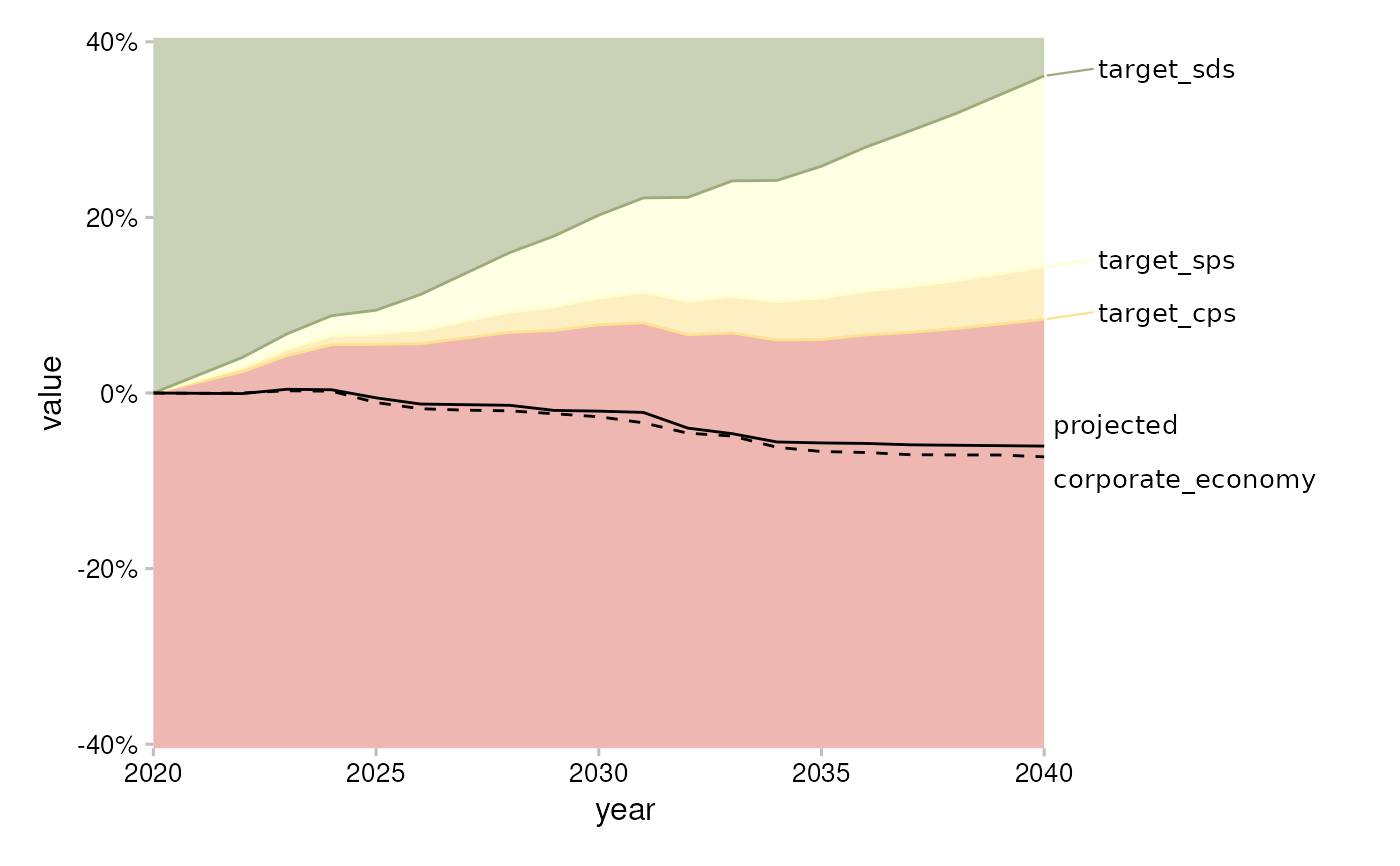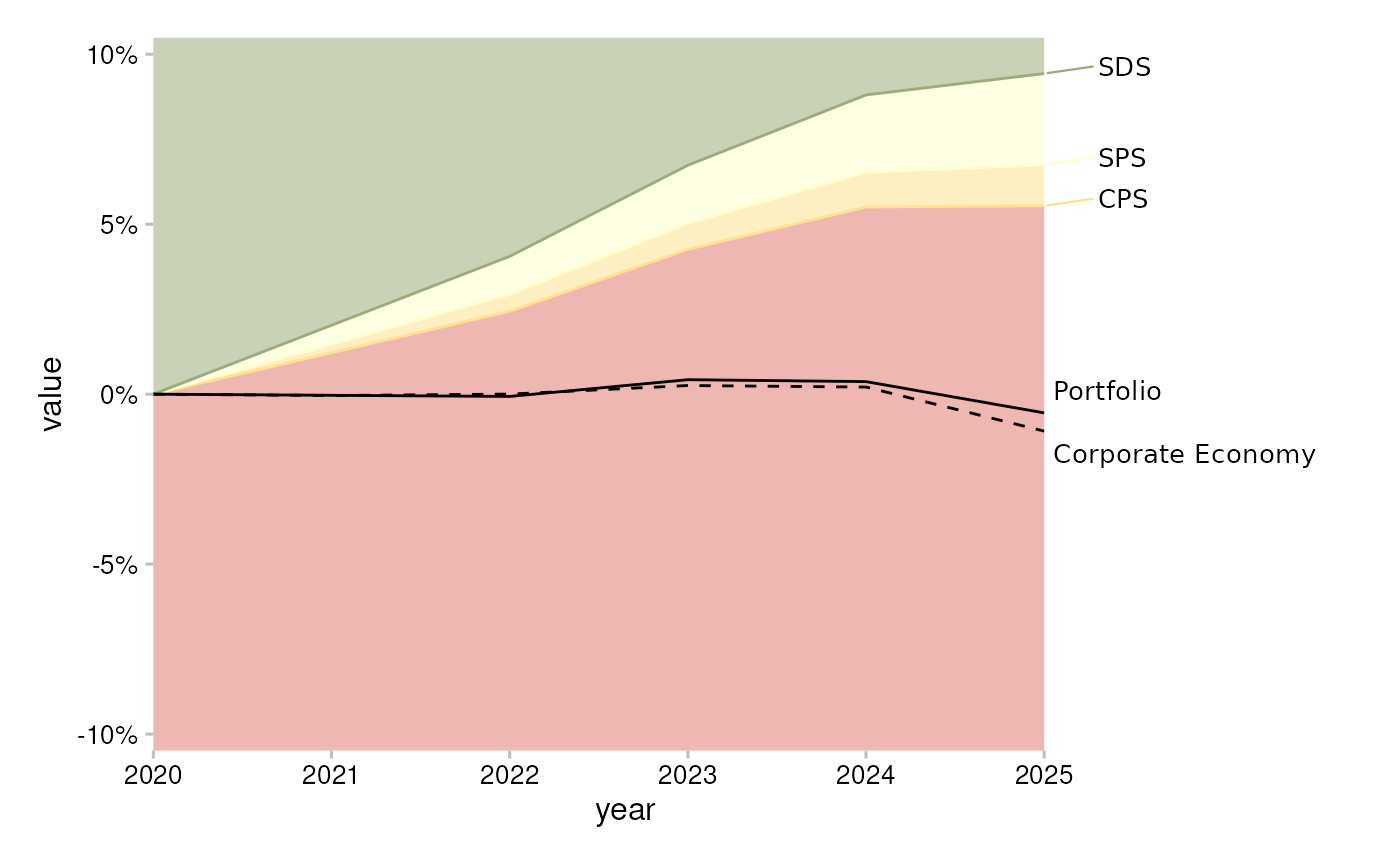Create a trajectory plot

## Usage

plot_trajectory(
data,
span_5yr = FALSE,
convert_label = identity,
center_y = FALSE,
value_col = "percentage_of_initial_production_by_scope",
perc_y_scale = FALSE
)

## Arguments

data

A data frame. Requirements:

• The structure must be like market_share.

• The following columns must have a single value: sector, technology, region, scenario_source.

• (Optional) If present, the column label is used for data labels.

span_5yr

Logical. Use TRUE to restrict the time span to 5 years from the start year (the default behavior of qplot_trajectory()), or use FALSE to impose no restriction.

convert_label

A symbol. The unquoted name of a function to apply to y-axis labels. For example:

• To convert labels to uppercase use convert_label = toupper.

• To get the default behavior of qplot_trajectory() use convert_label = recode_metric_trajectory.

center_y

Logical. Use TRUE to center the y-axis around start value (the default behavior of qplot_trajectory()), or use FALSE to not center.

value_col

Character. Name of the column to be used as a value to be plotted.

perc_y_scale

Logical. FALSE defaults to using no label conversion. Use TRUE to convert labels on y-axis to percentage using scales::percent (the default behavior of qplot_trajectory()).

## Value

An object of class "ggplot".

## Examples

# data must meet documented "Requirements"
data <- subset(
market_share,
sector == "power" &
technology == "renewablescap" &
region == "global" &
scenario_source == "demo_2020"
)

plot_trajectory(data)
#> Warning: The data argument of plot_trajectory() must be prepped already as of
#> r2dii.plot 0.4.0.
#> ℹ From the next release you will need to call
#>   r2dii.plot::plot_trajectory(data) prior to calling
#>   r2dii.plot::plot_trajectory().
#> ℹ Alternatively custom data preparation will also become possible.# plot with qplot_trajectory() parameters
plot_trajectory(
data,
span_5yr = TRUE,
convert_label = recode_metric_trajectory,
center_y = TRUE,
value_col = "percentage_of_initial_production_by_scope",
perc_y_scale = TRUE
)